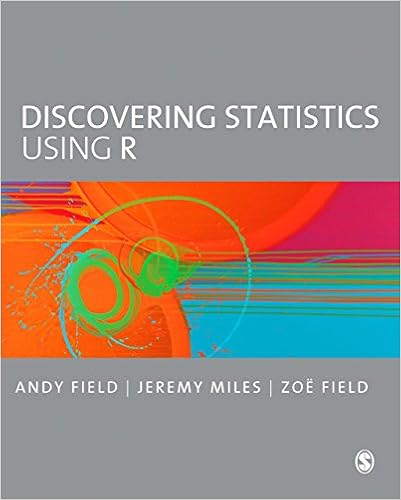https://github.com/profandyfield

Field, A. (2022). Discovering Statistics Using R and RStudio (2nd). SAGE. https://www.amazon.com/dp/B096B3F2H5/
Field, A. (2013). Discovering Statistics Using R. SAGE. https://www.amazon.com/dp/1446200469## Webcasts

For your visual delectation several webcasts are available that contain material relevant to the book. Most of these are actual lectures given by Andy Field, which cover the theory of some of the tests in the book.  Enjoy …!

These files are hosted on Andy Field’s YouTube channel, www.youtube.com/user/ProfAndyField and can therefore be viewed on any platform with any web browser. All you need is an internet connection. The contents of the website and webcasts have been prepared by Andy Field entirely and not SAGE Publications and does contain language and other content that some people may find offensive.

Tip: Click on each link to expand and view the content. Click again to collapse.

#### Chapter 10: Comparing several means: ANOVA (GLM 1)

Installing `discovr`

To use `discovr` you first need to installandand familiarise yourself withand good workflow practice. You can do this using this interactive tutorial. Once you have installedandyou can install `discovr`. The package is in development so you have to install it from github. To install the package execute (in):

```if(!require(remotes)){
install.packages('remotes')
}

remotes::install_github("profandyfield/discovr")```

If you are trying to install on a networked computer the install might fail (it’s to do install.packages not liking UNC paths, which I’m not even going to pretend to understand). The solution is to specify the location of yourlibrary at the point of install. Most networks will map network locations to a drive name (for example, at my own University, users accounts are on the ‘N’ drive). Find the location of yourlibrary (e.g., `N:/Documents/R/win-library/3.5`), possibly executing `.libPaths()` to help you, and specify this location using the `lib` argument:

`remotes::install_github("profandyfield/discovr", lib = "N:/Documents/R/win-library/3.5")`

## Interactive tutorials

I recommend working through this tutorial on how to install, set up and work withinandbefore starting the interactive tutorials.

### List of tutorials

• discovr_01: Key concepts in(functions and objects, packages and functions, style, data types, tidyverse, tibbles)
• discovr_02: Summarizing data (frequency distributions, grouped frequency distributions, relative frequencies, histograms, mean, median, variance, standard deviation, interquartile range)
• discovr_03: Confidence intervals: interactive app demonstrating what a confidence interval is, computing normal and bootstrap confidence intervals using, adding confidence intervals to data summaries.
• discovr_05: Visualizing data. The ggplot2 package, boxplots, plotting means, violin plots, scatterplots, grouping by colour, grouping using facets, adjusting scales, adjusting positions."
• discovr_06: The beast of bias. Restructuring data from messy to tidy format (and back). Spotting outliers using histograms and boxplots. Calculating z-scores (standardizing scores). Writing your own function. Using z-scores to detect outliers. Q-Q plots. Calculating skewness, kurtosis and the number of valid cases. Grouping summary statistics by multiple categorical/grouping variables.
• discovr_07: Associations. Plotting data with GGally. Pearson’s r, Spearman’s Rho, Kendall’s tau, robust correlations.
• discovr_08: The general linear model (GLM). Visualizing the data, fitting GLMs with one and two predictors. Viewing model parameters with broom, model parameters, standard errors, confidence intervals, fit statistics, significance, Bayes factors and Bayesian estimates (using default priors).
• discovr_09: Categorical predictors with two categories (comparing two means). Comparing two independent means, comparing two related means, effect sizes, robust comparisons of means (independent and related), Bayes factors and estimation (independent and related means).
• discovr_10: Moderation and mediation. Centring variables (grand mean centring), specifying interaction terms, moderation analysis, simple slopes analysis, Johnson-Neyman intervals, mediation with one predictor, direct and indirect effects, mediation using `lavaan`.
• discovr_11: Comparing several means. Essentially ‘One-way independent ANOVA’ but taught using a general linear model framework. Covers setting contrasts (dummy coding, contrast coding, and linear and quadratic trends), the F-statistic and Welch’s robust F, robust parameter estimation, heteroscedasticity-consistent tests of parameters, robust tests of means based on trimmed data, post hoc tests, Bayes factors.
• discovr_12: Comparing means adjusted for other variables. Essentially ‘Analysis of Covariance (ANCOVA)’ designs but taught using a general linear model framework. Covers setting contrasts, Type III sums of squares, the F-statistic, robust parameter estimation, heteroscedasticity-consistent tests of parameters, robust tests of adjusted means, post hoc tests, Bayes factors.
• discovr_13: Factorial designs. Fitting models for two-way factorial designs (independent measures) using both `lm()` and the `afex` package. This tutorial builds on previous ones to show how models can be fit with two categorical predictors to look at the interaction between them. We look at fitting the models, setting contrasts for the two categorical predictors, obtaining estimated marginal means, interaction plots, simple effects analysis, diagnostic plots, partial eta-squared and partial omega-squared, robust models and Bayes factors.
• discovr_14: Multilevel models.
• discovr_15: Repeated measures designs. Fitting models for one- and two-way repeated measures designs using the `afex` package. This tutorial builds on previous ones to show how models can be fit with one or two categorical predictors when these variables have been manipulated within the same entities. We look at fitting the models, setting contrasts for the categorical predictors, obtaining estimated marginal means, interaction plots, simple effects analysis, diagnostic plots, robust models and Bayes factors.
• discovr_16: Mixed designs. Fitting models for mixed designs using the `afex` package. This tutorial builds on previous ones to show how models can be fit with one or two categorical predictors when at least one of these variables has been manipulated within the same entities and at least one other has been manipulated using different entities. We look at fitting the models, setting contrasts for the categorical predictors, obtaining estimated marginal means, interaction plots, simple effects analysis, diagnostic plots, robust models and Bayes factors.
• discovr_18: Exploratory Factor Analysis (EFA). Applying factor analysis using the `psych` package. This tutorial uses a fictitious questionnaire (theAnxiety Scale, RAQ) with 23 items to show how EFA can be used to identify clusters of items that may, or may not, represent constructs associated with anxiety about using. We look at inspecting the correlation matrix, obtaining the Bartlett test and KMO statistics, using parallel analysis to determine the number of factors to extract, extracting factors, rotating the solution nd interpretation of the factors. We also learn to obtain Cronbach’s alpha on each of the subscales.